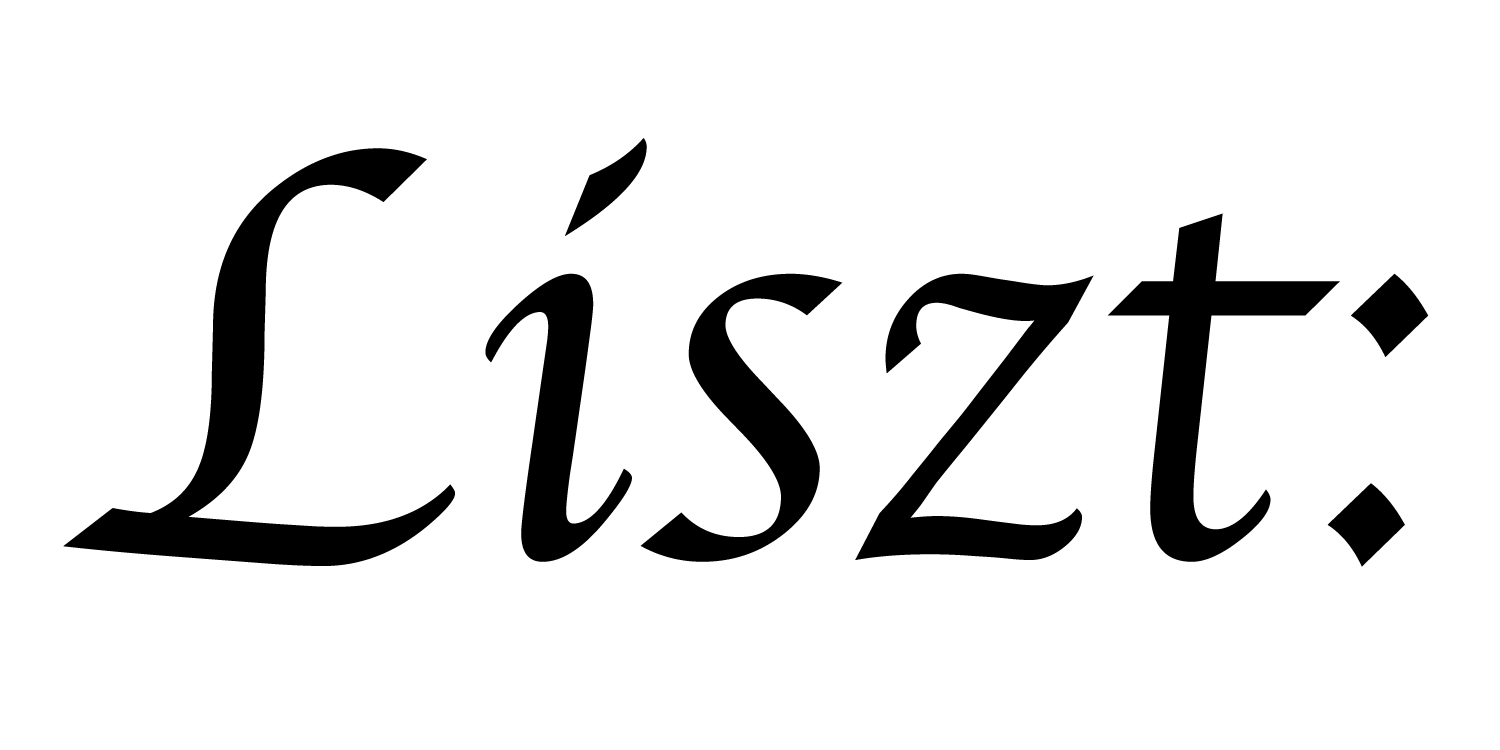# 04: User-defined Fields and Globals

We’re going to try to make the octahedron oscillate using a sinusoid here. This won’t really require simulating anything yet, but we’ll see how to define some data.

``````import 'ebb'
local L = require 'ebblib'

local ioOff = require 'ebb.domains.ioOff'
local PN    = require 'ebb.lib.pathname'
local mesh  = ioOff.LoadTrimesh( PN.scriptdir() .. 'octa.off' )

local vdb   = require('ebb.lib.vdb')
``````

The same start to our program.

``````mesh.vertices:NewField('q', L.vec3d):Load({0,0,0})
``````

Because `v.pos` stores the original position of the points, and because we want to remember that information, we’re going to have to define a new field to hold modified positions instead. Here we define that field on the vertices of the triangle mesh. This defines a `vec3d` quantity (a vector of 3 doubles) for each vertex. Then, we load in the initial value `{0,0,0}` everywhere to initialize the field.

``````local time = L.Global(L.double, 0)
``````

In order to control the oscillation, we’re going to define a global time variable. We give it type `double` and initial value `0`.

``````local ebb set_oscillation ( v : mesh.vertices )
v.q = 0.5*( L.sin(time) + 1) * v.pos
end

mesh.vertices:foreach(set_oscillation)
``````

Finally, we can define the oscillation function. It will take the original position of each vertex and scale it by a sinusoid-modulated amount, using the time as parameter to the sinusoid.

``````local ebb visualize ( v : mesh.vertices )
vdb.color({1,1,0})
vdb.point(v.q)
end
``````

And as before, we’ll want a simple visualization function. This time, we’ll plot the point coordinates from `v.q` rather than `v.pos` and we’ll set all the points’ colors to be yellow.

``````for i=1,360 do
for k=1,40000000 do end

time:set(i * math.pi / 180.0)
mesh.vertices:foreach(set_oscillation)

vdb.vbegin()
vdb.frame()
mesh.vertices:foreach(visualize)
vdb.vend()
end
``````

Finally, we’ll end this file with something that looks a bit more like a real simulation loop.

To start, we loop pointlessly for a long time in order to slow down the loop. Old videogames did this sometimes. Hacks with history.

Then, we’ll set the global variable using the current loop iteration, and use the set_oscillation function to compute the new vertex positions for the entire mesh.

Finally, we’ll wrap our visualization call in a few extra VDB calls to tell VDB to start a frame `vdb.vbegin()`, to clear the screen `vdb.frame()` and finally end the frame `vdb.vend()`.

Suppose you want to have the color oscillate together with the positions of the points. Try modifying the program to do that.

a part of the Liszt project and PSAAP II center at Stanford University